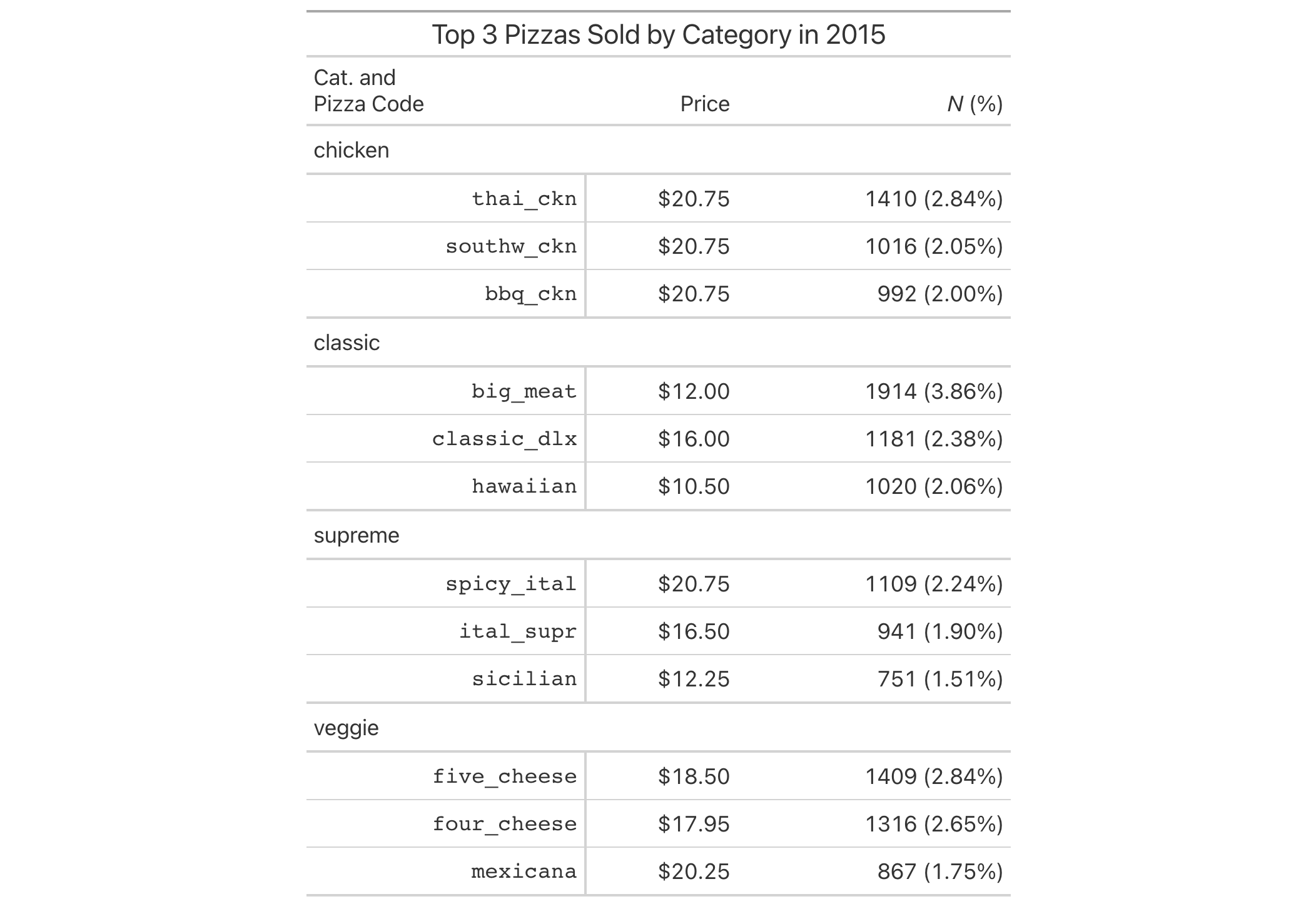# cols_merge_n_pct: Merge two columns to combine counts and percentages In gt: Easily Create Presentation-Ready Display Tables

 cols_merge_n_pct R Documentation

## Merge two columns to combine counts and percentages

### Description

The `cols_merge_n_pct()` function is a specialized variant of the `cols_merge()` function. It operates by taking two columns that constitute both a count (`col_n`) and a fraction of the total population (`col_pct`) and merges them into a single column. What results is a column containing both counts and their associated percentages (e.g., `⁠12 (23.2%)⁠`). The column specified in `col_pct` is dropped from the output table.

### Usage

``````cols_merge_n_pct(data, col_n, col_pct, rows = everything(), autohide = TRUE)
``````

### Arguments

 `data` A table object that is created using the `gt()` function. `col_n` A column that contains values for the count component. `col_pct` A column that contains values for the percentage component. This column should be formatted such that percentages are displayed (e.g., with `fmt_percent()`). `rows` Rows that will participate in the merging process. Providing `everything()` (the default) results in all rows in `columns` undergoing merging. Alternatively, we can supply a vector of row identifiers within `c()`, a vector of row indices, or a helper function focused on selections. The select helper functions are: `starts_with()`, `ends_with()`, `contains()`, `matches()`, `one_of()`, `num_range()`, and `everything()`. We can also use a standalone predicate expression to filter down to the rows we need (e.g., `⁠[colname_1] > 100 & [colname_2] < 50⁠`). `autohide` An option to automatically hide the column specified as `col_pct`. Any columns with their state changed to hidden will behave the same as before, they just won't be displayed in the finalized table.

### Value

An object of class `gt_tbl`.

### Comparison with other column-merging functions

This function could be somewhat replicated using `cols_merge()`, however, `cols_merge_n_pct()` employs the following specialized semantics for `NA` and zero-value handling:

1. `NA`s in `col_n` result in missing values for the merged column (e.g., `NA` + `⁠10.2%⁠` = `NA`)

2. `NA`s in `col_pct` (but not `col_n`) result in base values only for the merged column (e.g., `13` + `NA` = `13`)

3. `NA`s both `col_n` and `col_pct` result in missing values for the merged column (e.g., `NA` + `NA` = `NA`)

4. If a zero (`0`) value is in `col_n` then the formatted output will be `"0"` (i.e., no percentage will be shown)

Any resulting `NA` values in the `col_n` column following the merge operation can be easily formatted using the `sub_missing()` function. Separate calls of `sub_missing()` can be used for the `col_n` and `col_pct` columns for finer control of the replacement values. It is the responsibility of the user to ensure that values are correct in both the `col_n` and `col_pct` columns (this function neither generates nor recalculates values in either). Formatting of each column can be done independently in separate `fmt_number()` and `fmt_percent()` calls.

This function is part of a set of four column-merging functions. The other three are the general `cols_merge()` function and the specialized `cols_merge_uncert()` and `cols_merge_range()` functions. These functions operate similarly, where the non-target columns can be optionally hidden from the output table through the `hide_columns` or `autohide` options.

### Examples

Use `pizzaplace` to create a gt table that displays the counts and percentages of the top 3 pizzas sold by pizza category in 2015. The `cols_merge_n_pct()` function is used to merge the `n` and `frac` columns (and the `frac` column is formatted using `fmt_percent()`).

```pizzaplace |>
dplyr::group_by(name, type, price) |>
dplyr::summarize(
n = dplyr::n(),
frac = n/nrow(pizzaplace),
.groups = "drop"
) |>
dplyr::arrange(type, dplyr::desc(n)) |>
dplyr::group_by(type) |>
gt(
rowname_col = "name",
groupname_col = "type"
) |>
fmt_currency(price) |>
fmt_percent(frac) |>
cols_merge_n_pct(
col_n = n,
col_pct = frac
) |>
cols_label(
n = md("*N* (%)"),
price = "Price"
) |>
tab_style(
style = cell_text(font = "monospace"),
locations = cells_stub()
) |>
tab_header(title = "Top 3 Pizzas Sold by Category in 2015") |>
tab_options(table.width = px(512))
```5-14

### Function Introduced

`v0.3.0` (May 12, 2021)

Other column modification functions: `cols_align_decimal()`, `cols_align()`, `cols_hide()`, `cols_label_with()`, `cols_label()`, `cols_merge_range()`, `cols_merge_uncert()`, `cols_merge()`, `cols_move_to_end()`, `cols_move_to_start()`, `cols_move()`, `cols_unhide()`, `cols_width()`